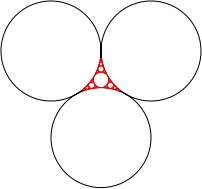## Sunday, October 21, 2007

### Drawing the Apollonian Gasket with Common Lisp and Vecto

In this post I'm going to show a simple, yet incomplete, program to draw the Apollonian Gasket using Common Lisp and Vecto.

The Wikipedia entry for the Apollonian Gasket defines it as:

In mathematics, an Apollonian gasket or Apollonian net is a fractal generated from three circles, any two of which are tangent to one another. It is named after Greek mathematician Apollonius of Perga.

Basically the Apollonian gasket is generated by finding the 4th circle that is tangent to a given tree tangent circles and repeating the process recursively.

According to Wikipedia in order to find the position and the radius of the 4th circle we use the Descartes theorem which implies that we can apply the following formula:Given that kn is the curvature of the circle n. The curvature can be calculated as 1/radius of the circle.

According to the Wikipedia article this formula could also be used to determine the position of the 4th circle. By multiplying each curvature value kn with a complex number that represents the position of the circle we can obtain a complex number with the position of the remaining circle.

For example given the following circles:We can calculate the position of the 4th circle (shown in red) by using the following function:

``(defun solve-equation (k1 k2 k3)   (let ((equation            (lambda (op)                 (+ k1 k2 k3                    (funcall op                        (* 2 (sqrt (+ (* k1 k2)                                      (* k2 k3)                                      (* k1 k3) ))))))))       (values (funcall equation #'+)               (funcall equation #'-))))``

This function is used to solve the equation shown above. We use values to return multiple values implied by the solution of the equation . For this post I'm only using the first solution (that's why the program is incomplete!!!).

To find the radius we can say:

``* (* 50 (solve-equation 1/50 1/50 1/50))6.4641013``

To find to position we use the complex numbers provided by Common Lisp and the same function defined above:

``* (defvar radius  (* 50 (solve-equation 1/50 1/50 1/50)))RADIUS* (* radius  (solve-equation (* 1/50 #c(50 50)) (* 1/50 #c(150 50)) (* 1/50 #c(100 136.6))))#C(83.56915 65.90832)``

In order to draw the picture of the Apollonian gasket we use the Vecto library which provides an easy way for drawing the picture.

``(defun draw-forth-circle (pos1 r1 pos2 r2 pos3 r3)     (set-rgb-stroke  1 0 0)     (let* ((curv1 (/ 1 r1))            (curv2 (/ 1 r2))            (curv3 (/ 1 r3))            (kz1 (* curv1 pos1))            (kz2 (* curv2 pos2))            (kz3 (* curv3 pos3))            (r4   (/ 1 (solve-equation curv1 curv2 curv3)))            (pos4 (* r4 (solve-equation kz1 kz2 kz3))))          (progn             (centered-circle-path                     (realpart pos4)                     (imagpart pos4)                     r4)             (stroke)             (list pos4 r4))))(defun draw-apollonian-gasket (c1 r1 c2 r2 c3 r3 steps)    (if (> steps 0)        (let ((lc41 (draw-forth-circle c1 r1 c2 r2 c3 r3)))             (draw-apollonian-gasket c1 r1 c2 r2 (car lc41) (cadr lc41) (- steps 1))             (draw-apollonian-gasket c2 r2 c3 r3 (car lc41) (cadr lc41) (- steps 1))             (draw-apollonian-gasket c3 r3 c1 r1 (car lc41) (cadr lc41) (- steps 1)))))(defun tria (file)   (with-canvas (:width 300 :height 300)     (set-rgb-stroke 1 1 1)     (set-line-width 1)     (translate 50  150)      (let* ((pos1 #c(50 50))            (r1   50)            (pos2 #c(150 50))            (r2   50)            (pos3 (complex                       100                      (- 50 (sqrt (- (* 100 100) (* 50 50))))))            (r3 50))          (centered-circle-path (realpart pos1) (imagpart pos1) r1)          (centered-circle-path (realpart pos2) (imagpart pos2) r2)          (centered-circle-path (realpart pos3) (imagpart pos3) r3)          (stroke)               (draw-apollonian-gasket                pos1 r1                pos2 r2               pos3 r3 5))     (save-png file)))``

Running this program generates the following picture:By applying `scale` and `translate` command we can obtain a better picture:This program only draws one portion of the complete Apollonian Gasket, in order draw the complete picture, the other solutions of the equation shown above must be used. In future posts I'm going to try to complete the program.